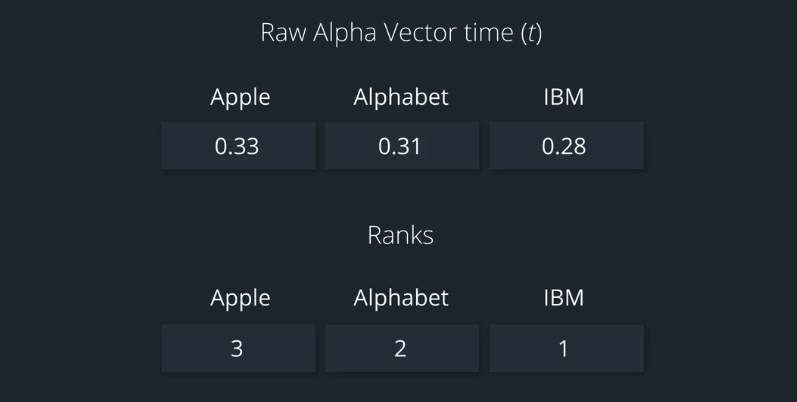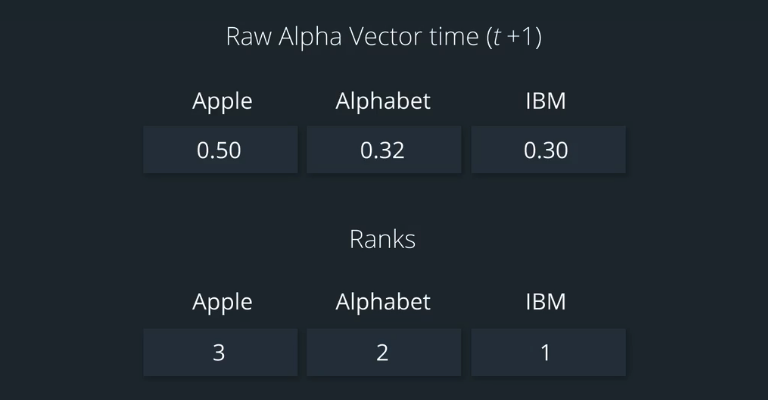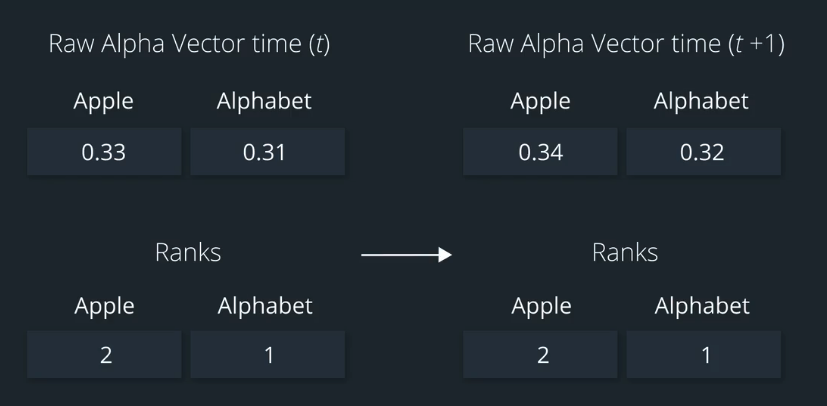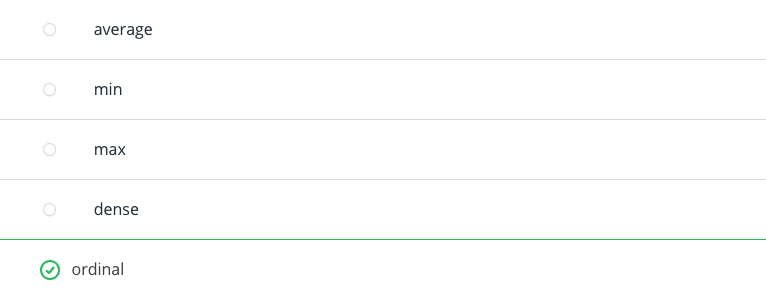# AI For Trading:Ranking (63)

You may notice, that if the amount we invest in each stock of our portfolio is tied to the Alpha value that we get from daily data, then we would be constantly buying and selling every day in order to follow the signal faithfully.

In other words, as the Alpha vector changes every day, we'd have to adjust our portfolio weights every day also.
We also have to address what happens to our Alpha vector when we encounter outliers, or extreme values in the data.

If we have had a large increase in the office signal for one stock,then a sharp decrease the next day, this would effectively tell us to buy a lot of that stock and then sell a lot of that stock the next day. This may or may not be warranted.

In the real world, trading costs money. So, we want to be very confident that if we go to make a trade and bear that cost, that it is indeed warranted. Some ways to keep extreme values from leading to unnecessarily large trades, are by clipping very large and small values at for example the 95th percentile and the fifth percentile.

This process is called winsorizing. Here's an example of winsorizing in Alpha vector which has Alpha values for each stock, for a single day. For any number that exceeds the 95th percentile, we've replaced that outlier with a number at the 95th percentile.

Also for any values that are below the fifth percentile, we replace those with a number at the fifth percentile. Again, this is called winsorizing. We can also deal with outliers, by setting a maximum magnitude allowed waits for any single stock.

Note that we would handle outliers for each Alpha vector which may be updated each day. Even when we've dealt with outliers, there's still the issue of whether it makes sense to buy and sell based on the signal if their relative magnitudes for the Alpha values don't change.

Let's again take the example of Apple and Alphabet. One day, Apple's Alpha value is 0.33 and Alphabet's value is 0.31. What if on the next day, Apple's Alpha value increased by 0.01 and Alphabet's Alpha value also increased by 0.01. If we translated these directly into portfolio weights, the weights would change slightly from day one today two.
But the important thing to notice is that we'd still be putting more money on Apple relative to Alphabet.

So, maybe we wouldn't actually want to change our positions at all. Often what we want to do, is have a more robust version of the signal which is able to withstand outliers, handle noise in the data, and also keep us from making potentially excessive traits. We can handle this with a ranking.

## Ranking 2

Ranking is a broadly useful method in statistics to make calculations more robust and less sensitive to noise.
So, how do we use ranking.## Ranking in Zipline

### Explore the rank function

The Returns class inherits from zipline.pipeline.factors.factor.
The documentation for rank is located hereand is also pasted below:

rank(method='ordinal', ascending=True, mask=sentinel('NotSpecified'), groupby=sentinel('NotSpecified'))[source] Construct a new Factor representing the sorted rank of each column within each row.

Parameters:

method (str, {'ordinal', 'min', 'max', 'dense', 'average'}) – The method used to assign ranks to tied elements. See scipy.stats.rankdata for a full description of the semantics for each ranking method. Default is ‘ordinal’.

ascending (bool, optional) – Whether to return sorted rank in ascending or descending order. Default is True.

mask (zipline.pipeline.Filter, optional) – A Filter representing assets to consider when computing ranks. If mask is supplied, ranks are computed ignoring any asset/date pairs for which mask produces a value of False.

groupby (zipline.pipeline.Classifier, optional) – A classifier defining partitions over which to perform ranking.

Returns:
ranks – A new factor that will compute the ranking of the data produced by self.

Return type:
zipline.pipeline.factors.Rank

By looking at the documentation, and the link to scipy.stats.rankdata (also pasted below), which option for parameter method would we choose if we want unique ranks associated with each stock, even when the values are tied?

Note When the documentation refers to "tied" values, it means instances where there are two alpha values for two different assets that are the same number, so there are different ways to handle the "tied" values when converting those values into ranks.

‘average’: The average of the ranks that would have been assigned to all the tied values is assigned to each value.

‘min’: The minimum of the ranks that would have been assigned to all the tied values is assigned to each value. (This is also referred to as “competition” ranking.)

‘max’: The maximum of the ranks that would have been assigned to all the tied values is assigned to each value.

‘dense’: Like ‘min’, but the rank of the next highest element is assigned the rank immediately after those assigned to the tied elements.

‘ordinal’: All values are given a distinct rank, corresponding to the order that the values occur in a.

By looking at the documentation, and scipy.stats.rankdata, which option for parameter method would we choose if we want unique ranks associated with each stock, even when the values are tied?Assuming a stock universe of 100 stocks, when a higher raw alpha value indicates a higher return, we have rank 1 for the most negative alpha value, and 100 for the most positive alpha value. When a lower raw alpha value indicates a higher return, which parameter can you use to choose a rank of 100 for the most negative value and 1 for the most positive value?

A: ascending = True
B: ascending = False